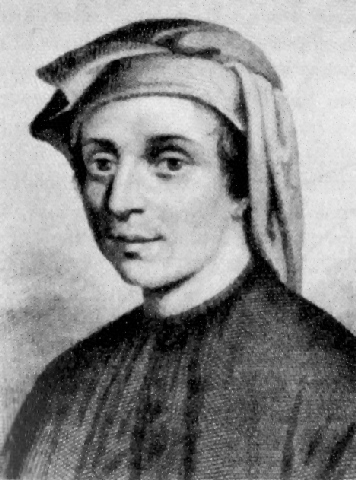## Posts

Showing posts from 2018

### Partial sums of arithmetical functionsIn this post we're going to look at some very interesting generalized formulas for computing the partial sums of some arithmetical functions .

### Continued fraction factorization methodThe factorization method that we'll discuss in this post, it's called the  continued fraction factorization method  (CFRAC), and is quite an old method, but still pretty interesting, sharing many concepts and ideas with other modern factorization methods.

### Curiosities in number theoryIn this post I would like to present some interesting exercises in number theory , along with some curious formulas and identities for some number-theoretic functions .

### Investigating the Fibonacci numbers modulo mThe  Fibonacci sequence  is, without doubt, one of the most popular sequences in mathematics and in popular culture, named after Italian mathematician Leonardo of Pisa (also known as  Fibonacci , Leonardo Bonacci, Leonardo of Pisa, Leonardo Pisano Bigollo, or Leonardo Fibonacci), who first introduced the numbers in Western European with his book  Liber Abaci , in 1202.